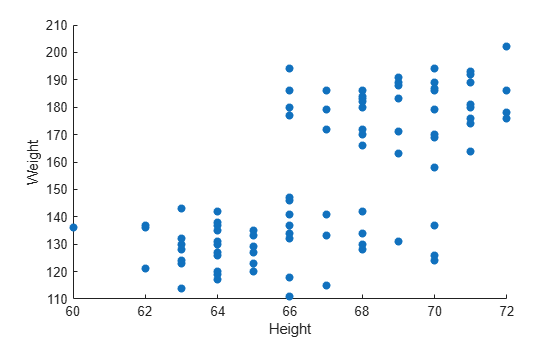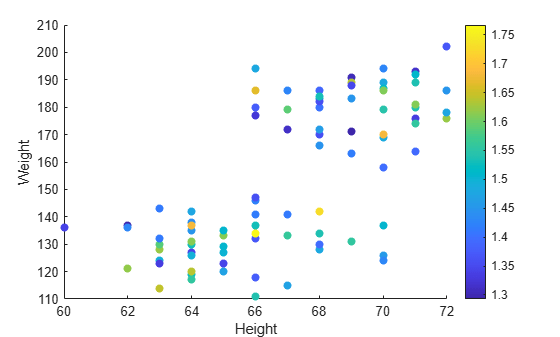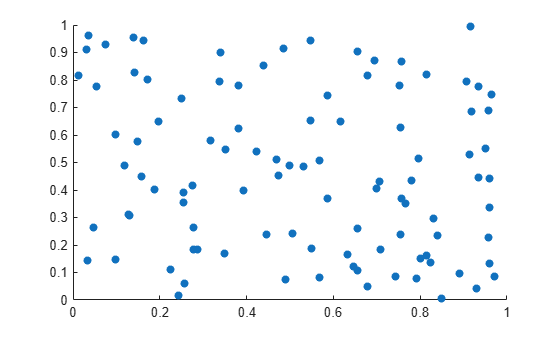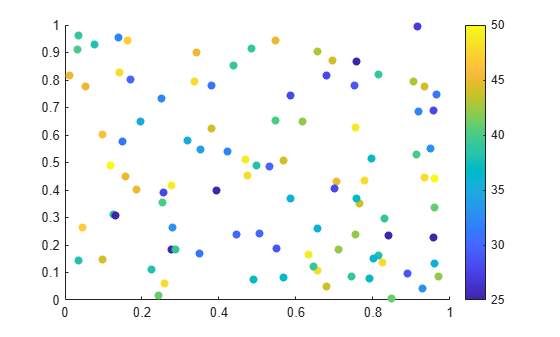Plots That Support Tables

Many plotting functions can plot data directly from a table. You pass the table as the first argument to the function followed by the variables you want to plot. You can specify either a table or a timetable, and in many cases, you can plot multiple data sets together in the same axes.

The following examples use the scatter and bubblechart functions to demonstrate the overall approach for plotting data from a table. To learn if a specific plotting function supports tables, refer to the documentation for that function.

Create Scatter Plot from a Table

Read patients.xls as a table and plot the Diastolic variable versus the Systolic variable. By default, the axis labels are automatically set to the variable names.

figure
scatter(tbl,"Systolic","Diastolic")Plot both blood pressure measurements against the Weight variable and specify filled markers. Then add a legend. By default, the plot uses different colors for the systolic and diastolic measurements, and the legend labels display the variable names.

Because the y-coordinates come from two different table variables, it is not clear what the y-axis label should be, so the label remains blank.

scatter(tbl,"Weight",["Systolic","Diastolic"],"filled")
legendUpdate Plot by Modifying the Table

When you pass a table to a plotting function, a copy of the table is stored in the SourceTable property of the plot object. If you change the contents of the table stored in that property, the plot is automatically updated to show the changes.

Read patients.xls as a table and plot the Weight variable versus the Height variable. Return the Scatter object as s, so you can access its properties later.

figure
s = scatter(tbl,"Height","Weight","filled");To change a value in the table, use dot notation to reference the table from the SourceTable property of the Scatter object. In this case, find the maximum value of the Weight variable and change it to 300. The plot is automatically updated.

Note: Making changes to a table in your workspace has no effect on the plot.

[~,idx] = max(s.SourceTable.Weight);
s.SourceTable.Weight(idx) = 300;Customize Marker Appearance with Table Variables

For some plots, you can vary the color, size, and transparency of the markers in your plot according to certain variables in your table. Some functions have arguments for specifying those aspects. For example, create a bubble chart of the patients data set. Plot the Weight variable versus the Height variable. Use the fourth argument to vary bubble sizes according to the Diastolic variable. Use the fifth argument to vary the color according to the Age variable.

Add a colorbar and resize the bubbles so that they range between 5 and 20 points.

figure
bubblechart(tbl,"Height","Weight","Diastolic","Age")

% Add a colorbar and resize the bubbles
colorbar
bubblesize([5 20])When you use the scatter function, you can use name-value arguments or set properties on the Scatter object to control different aspects of the markers. For example, create a scatter plot with filled markers, and set the ColorVariable property by specifying a name-value argument. Then add a colorbar.

figure
s = scatter(tbl,"Height","Weight","filled","ColorVariable","Age");
colorbarNext, vary the transparency of the markers according to the Systolic variable. To do this, you must also set the MarkerFaceAlpha property to 'flat'.

s.AlphaVariable = "Systolic";
s.MarkerFaceAlpha = "flat";Plot Table Coordinates with Scalar Marker Size

To specify a scalar marker size when creating a scatter plot from a table, use the SizeData name-value argument.

For example, read patients.xls as a table and plot the Weight variable versus the Height variable. Use the SizeData name-value argument to specify 200-point markers.

figure
scatter(tbl,"Weight","Height","SizeData",200)Combine Table and Vector Data

Many plots that support tables allow you to specify some aspects of your plot using a table variable and other aspects using vectors or matrices. For example, you can create a scatter plot using coordinates from a table and customize the colors of the markers by setting the CData property to a vector, an RGB triplet, or a matrix of RGB triplets.

Create a scatter plot using data from a table. Read patients.xls as a table, and plot the Weight versus the Height variables.

figure
s = scatter(tbl,"Height","Weight","filled");Next, change the colors of the plotted points using a vector. When you combine data from different sources like this, the size of each vector, matrix, or table variable must be compatible with the plot you are creating. In this case, create a vector called bpratio by dividing the systolic values by the diastolic values from the table. Because bpratio is derived from the same table as the Height and Weight variables, it has the same number of elements as those variables, and so it is compatible with this plot.

Color each point according to the blood pressure ratio by setting the CData property to bpratio. Then add a colorbar.

% Vary the color by blood pressure ratio
bpratio = tbl.Systolic./tbl.Diastolic;
s.CData = bpratio;

colorbarYou can also plot vectors or matrices, and modify the plot using table variables. After you create the plot, set the SourceTable property, and then set the table-related properties that you want. Table-related properties typically have the word Variable in their names. For example, plot two vectors of 100 random numbers.

x = rand(100,1);
y = rand(100,1);
figure
s = scatter(x,y,"filled");Change the marker colors so that they vary according to the values in a table variable. Read patients.xls as the table tbl. Set the SourceTable property and vary the marker colors according to the Age variable in the table. Because the table has 100 rows, and the plot has 100 points, the Age variable is compatible with the plot. Then, add a colorbar to the plot.

% Set source table and vary color by age
s.SourceTable = tbl;
s.ColorVariable = "Age";

colorbarNote: Standalone visualizations such as heatmap do not support combinations of table and vector data.